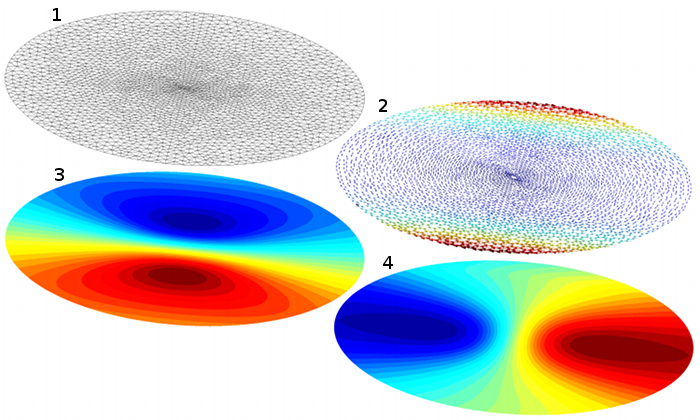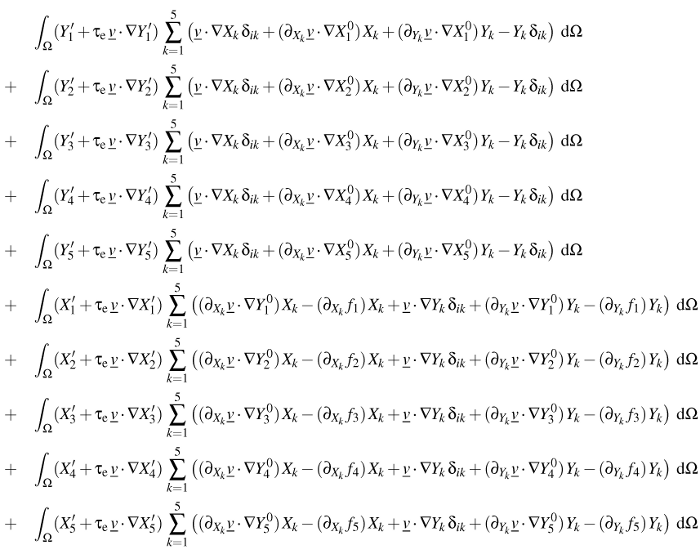#Metafor

ULiege - Aerospace & Mechanical Engineering

### Site Tools

team:gdeliege:nnm

## Nonlinear normal modes

#### Background

Nonlinear normal modes (NNMs) are an extension of linear normal modes to nonlinear systems. This is one of the research topics of the Space Structures & Systems Lab. (Prof. Kerschen). Actually, I knew nothing of the subject until Ludovic Renson told me about it and about the nonlinear equations he wanted to solve with finite elements. The idea to be faced with an unusual set of PDEs was too much of a temptation and I decided to implement a simple case in my old code as a test.

#### Problem description

Rigorous definitions of NNMs can be found in references [1-2]. Here, let us consider a discrete mechanical systems with $N$ degrees of freedom. A pair of state variables $u=x_k$ and $v=y_k$ is arbitrarily chosen among the displacements $x_i(t)$ and velocities $y_i(t)$, $i\in\{1,...,N\}$. The time variable can be eliminated and the state equations are rewritten as a set of 2N-2 partial differential equations, $$\begin{eqnarray*} v\, \partial_u X_i +f_k\, \partial_v X_i &=& Y_i \\ v\, \partial_u Y_i +f_k\, \partial_v Y_i &=& f_i \\ i\in\{1,...,N\},\; i&\neq& k \end{eqnarray*}$$ The unknowns are now space variables $X_i(u,v)$ and velocities $Y_i(u,v)$. The $f_i(u,v,X_j,Y_j),\; j\neq k$ are elastic and dissipative forces in the equations of motion. These equations are solved in a two-dimensional invariant manifold in phase space.

It turns out that the best way to solve these equations with finite elements is to define a pseudo-velocity $\vec{v}=[v, f_k]$ and to use a classical upwinding technique (Streamline Upwind Petrov-Galerkin formulation) to deal with the oscillations induced by the convective terms. The formulation reads :
Find $X_i\in V$ et $Y_i\in V$, $i=\{1, \ldots, N\}$, $i\neq k$, such that $$\begin{eqnarray*} \int_\Omega (Y'_i+\tau_{\rm e}\, \vec{v}\cdot\nabla Y'_i)\, (\vec{v}\cdot\nabla X_i-Y_i) \;{\rm d}\Omega = 0\;, && \forall Y'_i \in V \;, \\ \int_\Omega (X'_i+\tau_{\rm e}\, \vec{v}\cdot\nabla X'_i)\, (\vec{v}\cdot\nabla Y_i-f_i) \;{\rm d}\Omega = 0\;, && \forall X'_i \in V \;, \\ V = \{ f(u,v)\in H^1(\Omega):\, f(0,0)=0\} \;. && \end{eqnarray*}$$ The domain boundary must be tangent to the velocity field to avoid problems with the definition of boundary conditions. Ludovic came up with clever and effective solutions to deal with this issue . Fig. 1 shows results I obtained with my code for a simple 2-DOF system, also described inFigure 1. Finite element solution of a 2-DOF conservative system, calculated with my own code (mesh and visualization by Gmsh): (1) mesh of the elliptical domain, (2) pseudo-velocity field, (3) displacement $X2$, (4) velocity $Y2$.

I also attempted to solve a 6-DOF system corresponding to a cantilever beam. This requires the solution of a system of 10 equations with 10 unknown fields. Although my code has been designed to allow the definition of an arbitrary number of unknown fields, I had never tried more than 2 or 3 fields coupled in one single formulation. The Jacobian matrix for the Newton-Raphson algorithm is written below; I wrote a Python script to define this formulation in my code avoiding copy-paste errors. To my own surprise, it worked, although efficiency issues allowed me to solve the system on a small domain only.L. Renson, G. Deliége, G. Kerschen. An effective finite-element-based method for the computation of nonlinear normal modes of nonconservative systems. Meccanica, vol. 49(8), pp. 1901-1916, 2014.
 L. Renson. Nonlinear Modal Analysis of Conservative and Nonconservative Aerospace Structures. PhD Thesis, Université de Liège, 2014.

Back to main page

### Page Tools